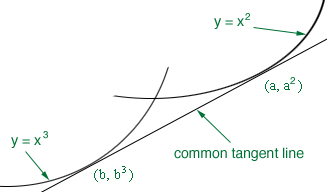Quandaries and Queries Hi, I was given the following problem and I tried equating the derivates but then I didnt know what to do from there. Problem: The x-axis is a common tangent of y = x2 and y = x3. Find the equation of another common tangent. Shanup Hi Shanup, You have two different curves and a common tangent line L. I drew such a diagram below with L tangent to y = x2 at x = a and L tangent to y = x3 at x = b.Find the slope of the tangent line to y = x2 at x = a and the slope of the tangent line to y = x3 at x = b. Equate these two expressions to get an equation relating a to b. Find the equation of the tangent line to y = x2 at x = a and the equation of the tangent line to y = x3 at x = b. These two expressions describe the same line so the y-intercepts must be the same. Equate the two expressions for the y-intercepts and you have another equation relating a to b. Solve the two equations for a and b. Penny Go to Math Central